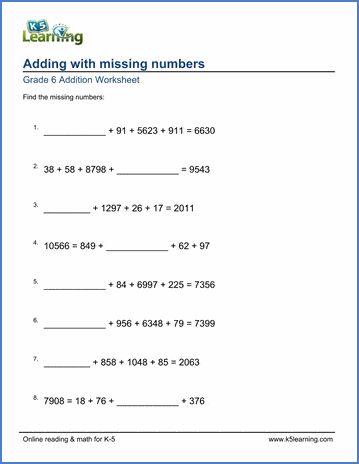Our grade 6 addition and subtraction worksheets focus on missing addend  & missing minuend/subtrahend problems with large numbers; essentially providing additional computational practice problems.

1,267+__+ 27 + 5 =1,862

## Subtraction

Missing minuend or subtrahend problems 171,490 - ___ = 23